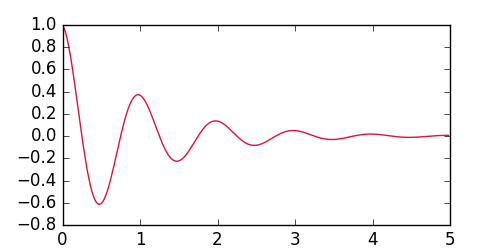Live Chat

If you don't want to do this, you can email us instead at contact@anvil.works.

Using Matplotlib with Anvil
« Anvil Cookbook
Using Matplotlib with Anvil

Matplotlib is a popular plotting library for Python. You may already be familiar with it, and want to use it in your Anvil apps. (If you’re not familiar with it, you should probably be using Anvil’s built-in Plotly support instead. If you do need to learn Matplotlib, start with this tutorial.)

We’re going to walk through a simple Matplotlib example that makes a graph like this:Server code

Matplotlib is available in Anvil’s server modules, if you choose the Full Python 2.7 or 3.7 runtime. Once you’ve made a plot, all you need to do is call `anvil.mpl_util.plot_image()` to capture that plot as an Anvil Media object.

You can then return that image from your server module, where you can display it on your page (with the `Image` component), or offer it for download (with the `Link` component), or even add it to a database.

Let’s see an example. Here’s the server function that makes our plot:

``````@anvil.server.callable
def make_plot():
# Make a nice wiggle
x = np.arange(0.0, 5.0, 0.02)
y = np.exp(-x) * np.cos(2*np.pi*x)

# Plot it
plt.figure(1, figsize=(10,5))
plt.plot(x, y, 'crimson')

# Return this plot as a PNG image
return anvil.mpl_util.plot_image()
``````

And here’s the client-side code to display that plot and make it downloadable:

``````    img = anvil.server.call('make_plot')

self.image_1.source = img